# Signal Sampling

View this topic in

# 3,226 resources related to Signal Sampling

### IEEE Organizations related to Signal Sampling

No organizations are currently tagged "Signal Sampling"

### Conferences related to Signal Sampling

No conferences are currently tagged "Signal Sampling"

### Periodicals related to Signal Sampling

No periodicals are currently tagged "Signal Sampling"

### Xplore Articles related to Signal Sampling

1963 IEEE International Solid-State Circuits Conference. Digest of Technical Papers, 1963

None

IEEE Transactions on Signal Processing, 1999

None

IEE Colloquium on Progress in Fibre Optic Sensors and Their Applications, 1995

Firstly, the theory of the white light interferometer is presented. This is followed by minimum peak height difference and related experimental results. The curve fitting signal processing scheme and the best order of polynomial for curve fitting are then discussed. Finally, displacement measurement with and without curve fitting signal processing is examined. The results show that, with a two wavelength ...

1996 IEEE International Conference on Acoustics, Speech, and Signal Processing Conference Proceedings, 1996

The paper presents a wavelet transform based frequency classifier for stochastic bandpass transient signals. Using compactly supported bases of wavelets, continuous nonstationary bandpass processes can be described by reduced dimension discrete time signal representations. We develop an optimization procedure to adapt both the sampling frequency and the discrete time wavelet filters to the continuous classes of signals involved. When compared ...

IEEE Signal Processing Letters, 2000

The classic equations for discrete time filter design by impulse invariance are not correct, in general. In particular, if the impulse response of the causal continuous-time filter is discontinuous at t=0, then the initial sample h of the discrete-time impulse response should equal one-half the value of the discontinuity, not the full value as classically given. Otherwise, the resulting frequency ...

### Educational Resources on Signal Sampling

#### IEEE.tv Videos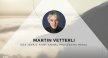Martin Vetterli accepts the IEEE Jack S. Kilby Signal Processing Medal - Honors Ceremony 2017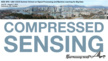Compressed Sensing: An Overview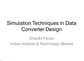SIMULATION TECHNIQUES FOR DATA CONVERTER DESIGN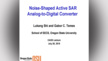Noise-Shaped Active SAR Analog-to-Digital Converter - IEEE Circuits and Systems Society (CAS) Distinguished Lecture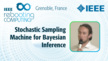Stochastic Sampling Machine for Bayesian Inference - Raphael Frisch at INC 2019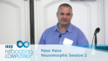Neuromorphic Mixed-Signal Circuitry for Asynchronous Pulse Processing Neuromorphic Mixed-Signal Circuitry for Asynchronous Pulse Processing - Peter Petre: 2016 International Conference on Rebooting Computing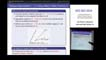Approximate Dynamic Programming Methods A Unified Framework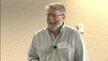Rebooting Computing: Randomness and Approximation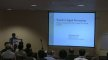ICASSP 2011 Trends in Design and Implementation of Signal Processing Systems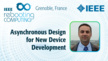Asynchronous Design for New Device Development - Laurent Fesquet at INC 2019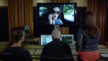Signal Processing and Machine Learning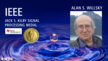Alan S. Willsky - IEEE Jack S. Kilby Signal Processing Medal, 2019 IEEE Honors Ceremony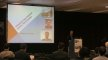ICASSP 2011 Trends in Multimedia Signal ProcessingICASSP 2011 Trends in Machine Learning for Signal Processing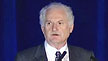ICASSP 2010 - New Signal Processing Application Areas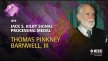2014 Jack S. Kilby Signal Processing Medal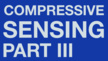The Fundamentals of Compressive Sensing, Part III: Sparse Signal Recovery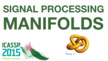Signal Processing on Manifolds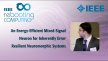An Energy-Efficient Mixed-Signal Neuron for Inherently Error Resilient Neuromorphic Systems - IEEE Rebooting Computing 2017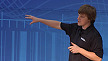IMS 2012 Microapps - System Simulation Featuring Signal Processing Blocks

#### IEEE-USA E-Books

• None

• None

• Firstly, the theory of the white light interferometer is presented. This is followed by minimum peak height difference and related experimental results. The curve fitting signal processing scheme and the best order of polynomial for curve fitting are then discussed. Finally, displacement measurement with and without curve fitting signal processing is examined. The results show that, with a two wavelength source, the central fringe can be identified if the sampling gap is smaller than the maximum sampling OPD gap. The noise in the system can be reduced by the curve fitting technique proposed. The best order of the polynomial used to carry out curve fitting is four according to the experimental results and the analysis.

• The paper presents a wavelet transform based frequency classifier for stochastic bandpass transient signals. Using compactly supported bases of wavelets, continuous nonstationary bandpass processes can be described by reduced dimension discrete time signal representations. We develop an optimization procedure to adapt both the sampling frequency and the discrete time wavelet filters to the continuous classes of signals involved. When compared to the classical Bayesian structures, the proposed receiver strongly reduces the required computational load. The receiver performance is accessed by Monte Carlo simulation, the probabilities of detection for each signal class, and the probability of false alarm, being computed.

• The classic equations for discrete time filter design by impulse invariance are not correct, in general. In particular, if the impulse response of the causal continuous-time filter is discontinuous at t=0, then the initial sample h of the discrete-time impulse response should equal one-half the value of the discontinuity, not the full value as classically given. Otherwise, the resulting frequency response does not equal the sum of shifted versions of the original response as expected. This effect will typically be seen only in low order filters such as first order lowpass filters or second order bandpass filters.

• Explicit formulas for estimating the frequency of a sinusoid in white noise are proposed. The accuracy of the estimate obtained by these formulas is very close to the Cramér-Rao bound in the case of very short sequences.

• In this paper, we propose a new theorem relating to the generalized spectrum of an interpolating function. In particular, our theorem provides an incite to design interpolants in frequency domain. We consider a more pragmatic situation where the reconstruction filter is non-ideal and occupies some extra bandwidth. The spectrum is shown to satisfy the orthogonality condition which the strongest constraint for the admissibility of an interpolant. Extending our formulation, we show that the spectrum of generating function (Sine) used in Shannon's sampling theorem is a special case of spectrum proposed in this paper.

• In the transversal plane CT exhibits a nearly rotational symmetric point spread function. Pixel sampling is typically done on Cartesian grids which are not ideal from a signal processing point of view. It is advantageous to use a hexagonal grid which can capture the same signal components with 13% fewer sampling points. In 3D one can even save 29%. We developed an efficient scheme to allow for arbitrarily shaped field of views and a hierarchical memory layout. The latter divides the images into small hexagonal subimages, similar to honeycombs, whose size is small enough to avoid cache misses on CPU-based algorithms or to be used on dedicated signal processing hardware such as GPUs (graphics processing units) or CBEs (cell broadband engines) that may be memory limited. As the final step a resampling algorithm converts from the hex domain to the Cartesian domain before storing images. We implemented a hyperfast CBE-based Feldkamp algorithm for the hexagonal lattice and compared its performance and its image quality to reconstructions using the standard Feldkamp algorithm. Both algorithms were run on the Dual Cell Based System (DCBS, Mercury Computer Systems, Berlin, Germany) on two CBEs with 3.2 GHz each. A 5123 volume was reconstructed from 720 projections of 5122 detector pixels. Image quality of the hexagonal approach was identical to the direct approach using the Cartesian lattice: the maximum relative difference between the MTFs was 1% and image noise differed by not more than 3%. The improvement in reconstruction speed was approximately 12% for the hexagonal grid which is slightly lower than the expectation. The complete reconstruction finished in about 10 s. With identical image quality reconstruction on the hexagonal grid is a simple and effective approach to significantly reduce memory consumption and reconstruction time. Existing reconstruction algorithms can be easily modified to operate in the hexagonal domain.

• The problem of sampling a two-dimensional signal at below the conventional Nyquist sampling density is considered. Although optimal sampling at below Nyquist density is possible through a process of periodic sample deletion, the resulting irregular sampling pattern has limited utility in real-world applications. In this paper, a novel algorithm is proposed to perform uniform sampling of the data at below Nyquist density, yet allows for perfect reconstruction of the signal. By exploring the special signal structure of sinograms in computer aided tomography, the technique is used for signal reconstruction from sinograms sampled at below Nyquist rate. Computer simulation results are presented to demonstrate the validity of the algorithm.<>

• In this paper, the design of two-dimensional (2-D) variable fractional delay FIR filter using 2-D differentiators is presented. First, the Taylor series expansion is used to transform the specification of a 2-D variable fractional delay filter into the one of a 2-D differentiator. This transform makes the design problem of a 2-D fractional delay filter reduce to the design of 2-D differentiators with different orders. Then, a simple window method is proposed to design the 2-D differentiators. Also an analysis of the design error is provided. Finally, examples are demonstrated to illustrate the effectiveness of this new design approach.